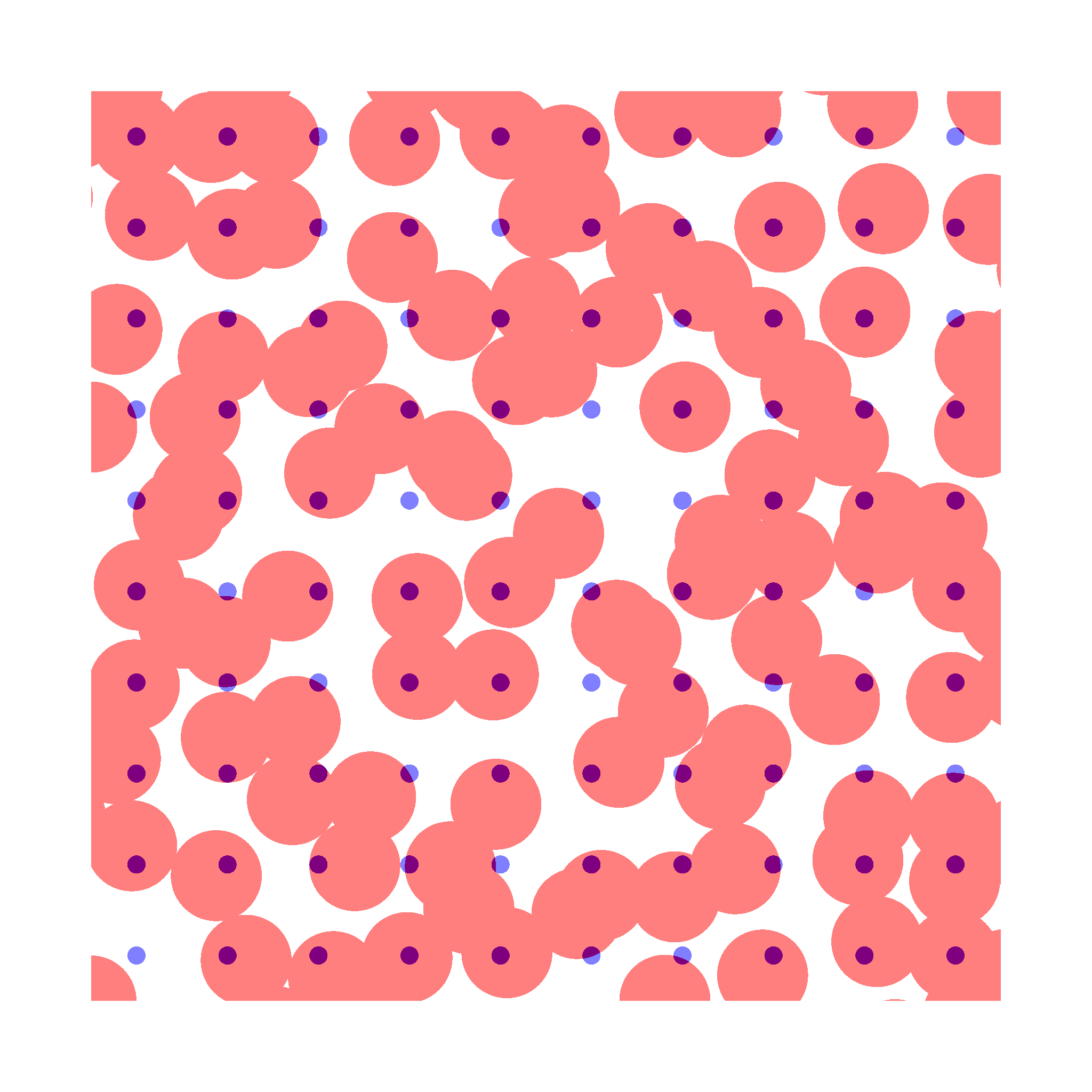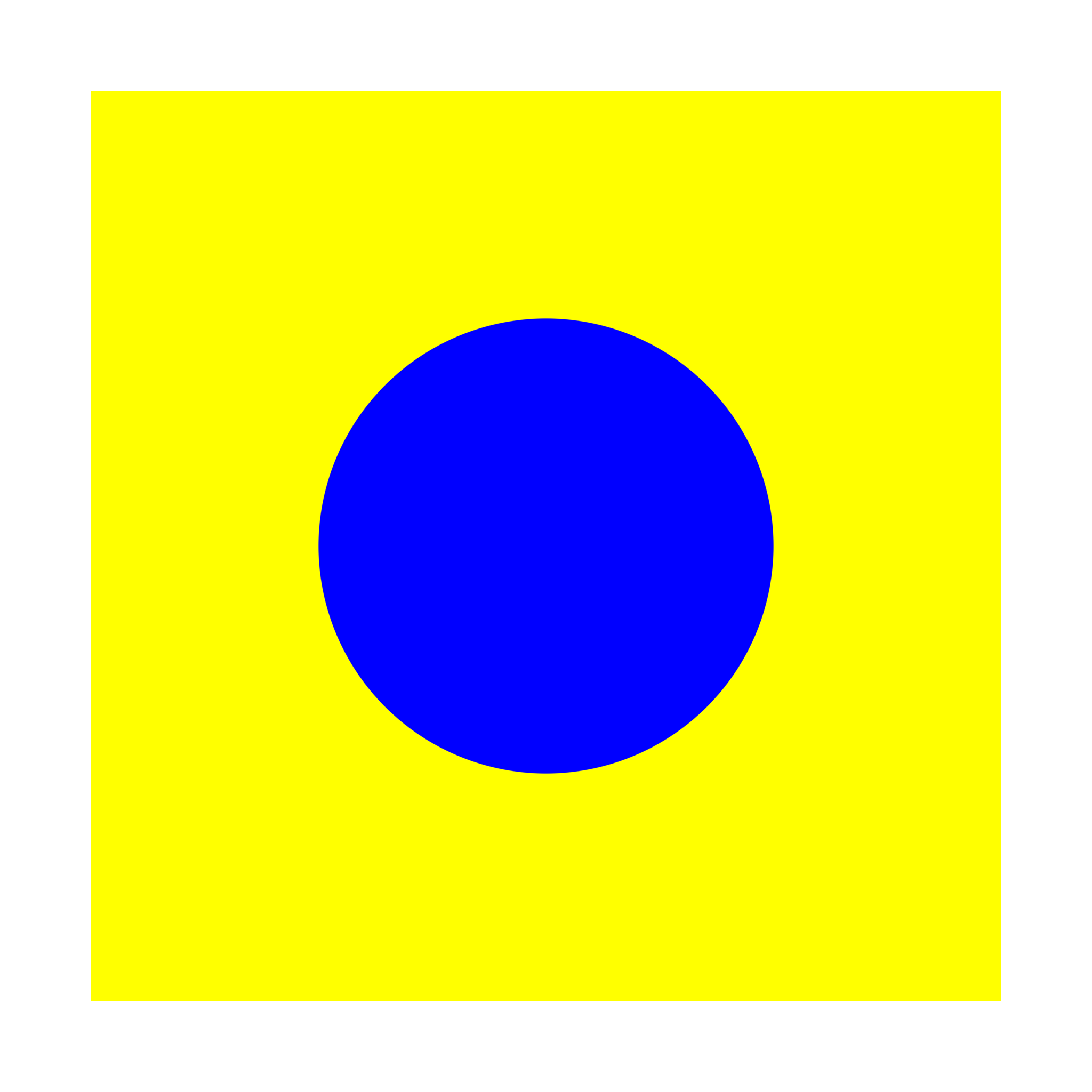Nearly Picked Makefiles

# npm

## glsl-simple-alpha-compositing

1.0.3 • Public • Published

# glsl-simple-alpha-compositing

GLSL implementation of the simple alpha compositing function, as described in the W3C Editor's draft in Compositing and Blending.

## Usage## An example: set the background color in a GLSL fragment shader.

The simple alpha compositing can be used to set the background colour in a glsl fragment shader.

The following code uses the `step` function to set the alpha channel value to 1 for all the points which distance from the circle centre is less than 0.25, otherwise, the alpha channel is set to 0.

The `simpleAlphaCompositing` function is used to compose the blue circle with a yellow background.

``````vec3 circleField (vec2 p) {
// Colors
vec3 circleColor = vec3(0., 0., 1.); // blue
vec3 backgroundColor = vec3(1., 1., 0.); // yellow

// Background field
vec4 background = vec4(backgroundColor, 1.);

// Foreground field, a circle.
vec2 center = vec2(.5);
float d = distance(p, center);
float delta = 0.0005;
// Note that the circular shape is implemented in the alpha channel.
vec4 circle = vec4(circleColor, smoothstep(d - delta, d + delta, 0.25));

return simpleAlphaCompositing(circle, background);
}

void main() {
vec3 color = circleField(vUv);
gl_FragColor = vec4(color, 1.0);
}
``````## Keywords

### Install

`npm i glsl-simple-alpha-compositing`

1

1.0.3